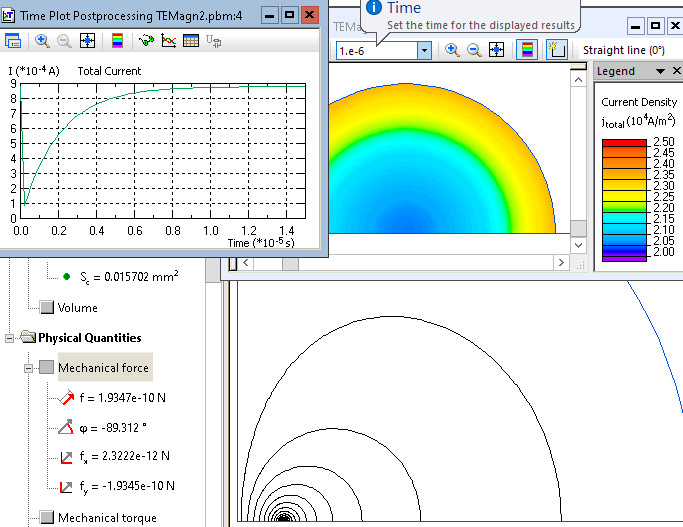QuickField

A new approach to field modelling
 Language: Global English Deutch Espanol Francais Italiano Danmark Ceske Chinese Pycckuü

Main >> Applications >> Sample problems >> Transient eddy currents in a two-wire line

# Transient eddy currents in a two-wire line

This is an example of the two-wire line simulation, performed with QuickField software.

Problem Type:
Plane problem of transient magnetics.

Geometry:

Transmission line consists of two copper conductors with equal but opposite currents. All dimensions are in millimeters.

Given:
Magnetic permeability of air μ = 1;
Magnetic permeability of copper μ = 1;
Conductivity of copper g = 56,000,000 S/m;
Voltage applied U = 0.001 V;

Calculate the transient currents within the conductors.

Solution:
The resistance of one conductor can be calculated as Rcond = l / (g · S),
where
S = πr2 - cross-section area of conductor,
l - length of the line.

Rcond = 1 / (56·106 · (π·0.00012)) = 0.5684 Ohm

The resistance of both conductors is R = 2·Rcond = 2·0.5684 = 1.1368 Ohm

The inductance of the transmission line can be calculated as L = μ0·l/π · (ln(D/r) + 0.25), where
D - distance between conductors.
L = 4π·10-7·1/π · (ln(0.02/0.0001) + 0.25) = 2.219·10-6 Hn

 The transient current for equivalent electric circuit with lumped parameter is described by the formula I(t) = U/R · (1 - e-t/T), where T=L/R - characteristic time of the circuit.Results:• View simulation report in PDF
• Download simulation files (files may be viewed using any QuickField Edition).